# Tactics for System I

## Tactics for System 1

##### 11/16/06

An unfinished derivation can be thought of as being a collection of 'facts' that you have and a collection of 'problems' that remain to be solved. The standard way of approaching a proof consists of extending the facts, essentially doing the proof in a forwards direction, until no problems remain. But it is also possible to do a proof, or parts of a proof, by looking at the problems, and reducing these to earlier problems, essentially doing the proof in a backwards direction, again until no problems remain.

Tactics are a means of allowing the User to adopt a flexible approach to a proof, by doing it forwards, backwards, or a mixture of both. Tactics are switched on from the Wizard menu (and they can be toggled on and off while a proof is in progress, if that is what the User wants).

Most of the Tactics are problem solving or backwards tactics. And these work with the current problem, that is with the next target line or the insertion point. Now, since this line is unique, there is no need to select it. So most the Tactics do not need any selection of lines in the proof, they just need to user to invoke the appropriate Menu item. Some Tactics, particularly fact extending ones, do need the selection of lines. Just which lines need to be selected is noted in the documentation below.

## The Tactics

### The Tactic of Assumption Ass

This behaves the same as the Rules.

It is unusual to make an assumption while using tactics (because that assumption can never be dropped)-- so this tactic is discouraged.  On rare occasions it is useful (consider how Fx,Ga ∴ (∀x)Ga is proved-- the problem here is that x is free in an assumption, although not in one that is actually used, and this excludes the use of Universal Generalization; the derivation is done by proving Ga ∴ (∀x)Ga and adding the assumption Fx last.)

### The Tactic for the Introduction of Negation ~I

This Tactic can be employed when the current target has the form ~<formula> , thus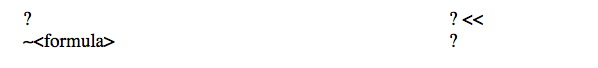That is, the formula you are trying to obtain has a ∼ as its main connective-- the formula you are trying to obtain has the form ∼<formula>.

The Tactic asks for the <positive horn>  of the contradiction. (It also gives you a bit of a hint here; it lists all candidate Positive Horns; you just have to choose the right one...) [Explanation of the terminology:- When you have a contradiction say A and ∼A , one of the formulas (the one without the negation in front) is known as the 'positive horn' of the contradiction, and the other, the 'negative horn'. So, to give a further example, with the contradiction ∼(∀x)∼Gx and (∀x)∼Gx, the positive horn is (∀x)∼Gx and the negative horn ∼(∀x)∼Gx.]

The program can be configured to use the propositional constant Absurd (this is the usual configuration for beginning logicians).

With Absurd in use, the program lays out all or part of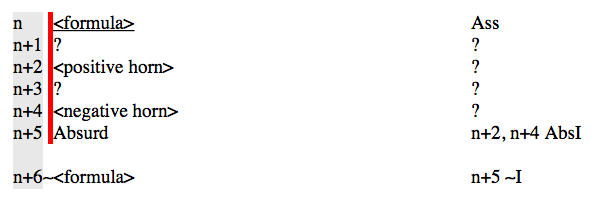It checks the facts. If <positive horn>  is available, then lines n+1 and n+2 are omitted (and the line numbers adjusted).  If <negative horn>  is available, then lines n+3 and n+4 are omitted (and the line numbers adjusted).

If Absurd is not in use, the program lays out all or part of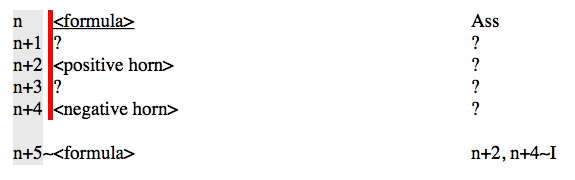It checks the facts. If <positive horn>  is available, then lines n+1 and n+2 are omitted (and the line numbers adjusted).  If <negative horn>  is available, then lines n+3 and n+4 are omitted (and the line numbers adjusted).

### The Tactic for the Elimination of Negation ∼E

There are two forms to this.

If there is a formula, starting with a double negation, among the body of the proof, and the User selects it, this tactic acts exactly like the standard rule (ie it removes the double negation).

If no formula or line is selected, and yet ∼E is chosen off the Rules Menu, this tactic acts as a problem reducing tactic and lays out the derivation as though the User had proved the last line by ∼E.

So the transformation is:

before the tactic ∼E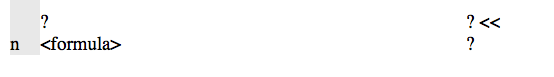after the tactic ∼E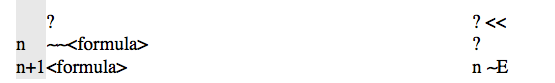### The Tactic for the Introduction of Conjunction ∧I

This Tactic can be employed when the current target has the form <formula1>∧<formula2> , thusIt displays all or part ofIt checks the facts. If <formula1>  is available, then lines n and n+1 are omitted (and the line numbers adjusted).  If <formula2>  is available, then lines n+2 and n+3 are omitted (and the line numbers adjusted).

### The Tactic for the Elimination of Conjunction ∧E

This behaves the same as the Rules (and so you need to select a conjunction from among the 'facts').

### The Tactic for the Introduction of Disjunction ∨I

This Tactic can be employed when the current target has the form <formula1>∨<formula2>, thus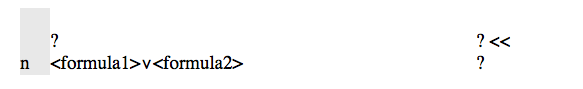It checks the facts. If either <formula1> or <formula2> is available, as line k, say, then it justifies the current target displaying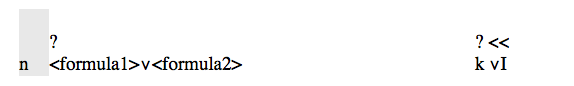and moves on to the next problem.

If neither are available it displays: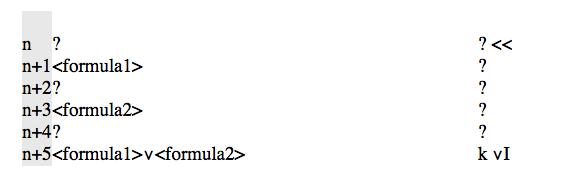The idea here is that you derive one of these and edit out the other one; you do not have to choose at this point which one you wish to prove. (If you are completely confident as to which you will prove, edit out the other straight away and click ∨I a second time.)

### Tactic for the Elimination of Disjunction ∨E

This Tactic can be employed with a selected disjunct formula, from among the facts, and any current target, thusIt displays all or part of: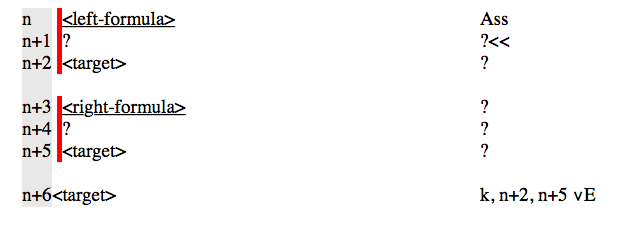It checks the facts. If <target> is available at the first stage, then lines n+1 and n+2 are omitted. If <target> is available at the second stage, then lines n+4 and n+5 are omitted.

### The Tactic for the Introduction of the Conditional ⊃I

This Tactic can be employed when the current target has the form <left-formula>⊃<right-formula> , thusIt displays all or part ofIt checks the facts. If <right-formula>  is available, then lines n+1 and n+2 are omitted (and the line numbers adjusted).

### The Tactic for the Elimination of the Conditional ⊃E

This behaves the same as the Rules.

### The Tactic for the Introduction of the BiConditional ≡I

This Tactic can be employed when the current target has the form <left-formula>≡<rightformula>, thus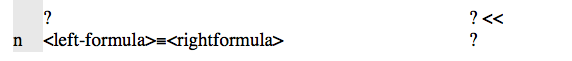It displays all or part of: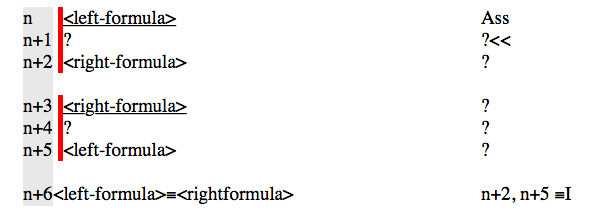It checks the facts. If <right-formula> is available at the first stage, then lines n+1 and n+2 are omitted. If <left-formula> is available at the second stage, then lines n+4 and n+5 are omitted.

### The Rule of Elimination of the BiConditional ≡E

This behaves the same as the Rules.

### The Rule of Introduction of the Universal Quantifier, Universal Generalization UG

This Tactic can be employed when the current target has the form (∀<variable>)<scope>, and <variable>is not free in the assumptions of the target line, thus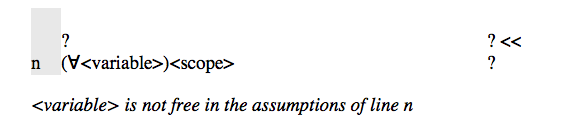If displays: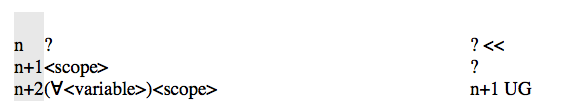It checks the facts. If <scope> is available, then lines n and n+1 are omitted.

### The Rule of Elimination of the Universal Quantifier, Universal Instantiation UI

This behaves the same as the Rules.

### The Tactic for the Introduction of the Existential Quantifier, Existential Generalization EG

This Tactic can be employed when both the current target has the form (∃<variable>)<scope> , thus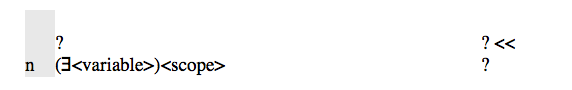You will be asked which <term> was generalized on and, provided the <variable> is free for the <term>  in the <scope>, matters will proceed.

It checks the facts. If <scope>  [<term>/<variable>]  is available, say at line k, then it justifies the current target displaying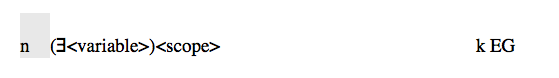and moves on to the next problem.

If <scope>  [<term>/<variable>] is not available it displays: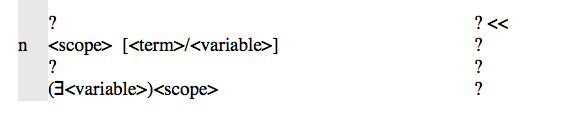The idea here is that you may not know which term was generalized on; you can make a guess and, perhaps, edit out later and make another guess... When you are able to derive <scope>  [<term>/<variable>] you merely have to use the Tactic for EG again to complete this step. (This is similar to the Tactic for ∨I.)

### The Tactic for the Elimination of the Existential Quantifier, Existential Instantiation EI

This Tactic can be employed with a selected existential formula (∃<variable>)<scope>, from among the facts, say at line k, and any current target, thuswhere the <variable> is not free either in the <target> or in the assumptions of the <target>, line n.

It displays all or part of: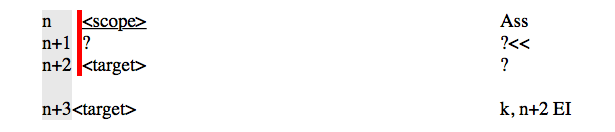It checks the facts. If <target> is available, then lines n+1 and n+2 are omitted.